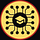# What is Seaborn?

`pip install seaborn`
`import seaborn as sns`
`data = sns.load_dataset("penguins")`
`data[:5]`
`data.shape#Output:(344, 7)`
`sns.set_theme()# For the image quality of the graphic. sns.set(rc={"figure.dpi":300})# For the size of the graphicssns.set(rc = {"figure.figsize":(6,3)})`

# 1- Scatter Plot

`sns.scatterplot( x = "bill_length_mm",                  y = "bill_depth_mm",                  data = data,                  hue = "species")`

# 2. Histogram

`sns.histplot(x = "flipper_length_mm", data = data)`
`sns.histplot(data=data, y="flipper_length_mm")`
`sns.histplot(data=data, x="flipper_length_mm", binwidth=3)`
`sns.histplot(data=data, x="flipper_length_mm", kde=True)`
`sns.histplot(data=data, x="flipper_length_mm", hue="species")`

# 3. Bar Plot

`sns.barplot(x = "species", y = "flipper_length_mm", data = data)`
`sns.barplot(x = "species",             y = "flipper_length_mm",             data = data,             hue = "sex")`

# 4. Box Plot

`sns.boxplot(x = "species", y = "flipper_length_mm", data = data)`
`sns.boxplot(x = "species",             y = "flipper_length_mm",             data = data,             hue = "sex")`

# 5. Violin Plot

`sns.violinplot(x = "species", y = "flipper_length_mm", data = data)`
`sns.violinplot(x = "species",                y = "flipper_length_mm",                data = data,                hue = "sex")`

# 6. Facet Grid

`sns.FacetGrid(data, col="island", row="sex")`
`sns.FacetGrid(data, col="island", row="sex").map(sns.histplot, "flipper_length_mm")`
`sns.FacetGrid(data, col="island", row="sex").map(sns.distplot, "flipper_length_mm")`

# 7. Pair Plot

`sns.pairplot(data, hue="species", height=3)`
`sns.pairplot(data, hue="species", diag_kind="hist")`

# 8. Heatmap

`sns.heatmap(data.corr())`
`sns.heatmap(data.corr(), annot=True)`

# Conclusion## Data Visualization with Python

11 stories--

--

## More from Geek Culture

A new tech publication by Start it up (https://medium.com/swlh).